# How to Evaluate Computer Vision Models with Confusion Matrix

We are excited to help you learn about the Confusion Matrix and how it can be used to evaluate and determine steps to improve your Computer Vision models.John Hoang
Editor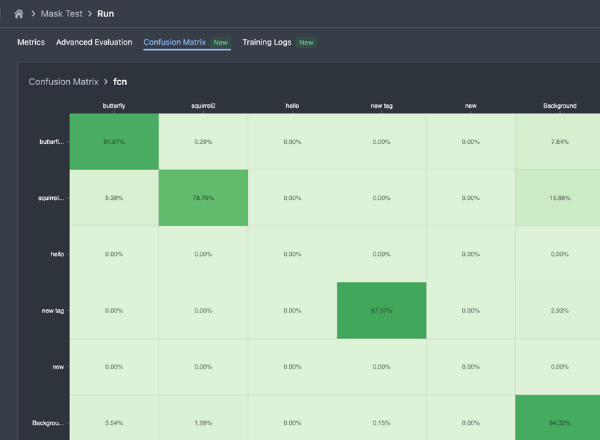##### CONTENTS

Evaluating the performance of deep learning models, especially in computer vision, is certainly not an easy task. While metrics like Average Recall, Average Precision, and mAP provide summary performance indicators, they often cannot capture the full picture. To supplement this dearth of information, the confusion matrix offers a more detailed view of the model’s behavior in its capability of classifying objects, by quantitatively highlighting where and what classification errors are being made by the model, thereby enriching our understanding of model performance.

## What is a Confusion Matrix?

A confusion matrix is a table used to visualize and quantify the performance of a classification algorithm by indicating where the algorithm classified a value as compared to the ground truth. In the case of computer vision, the simplest form of a classification algorithm is a classification model, which classifies images into user-defined labels.

Let’s better understand how confusion matrices are constructed by going through an example. The model below is a classification model being trained to classify whether the image contains a car, a truck, or a motorcycle. A typical model training process has evaluation epochs at regular intervals. During the evaluation epoch, we evaluate the model on a validation set of 300 images.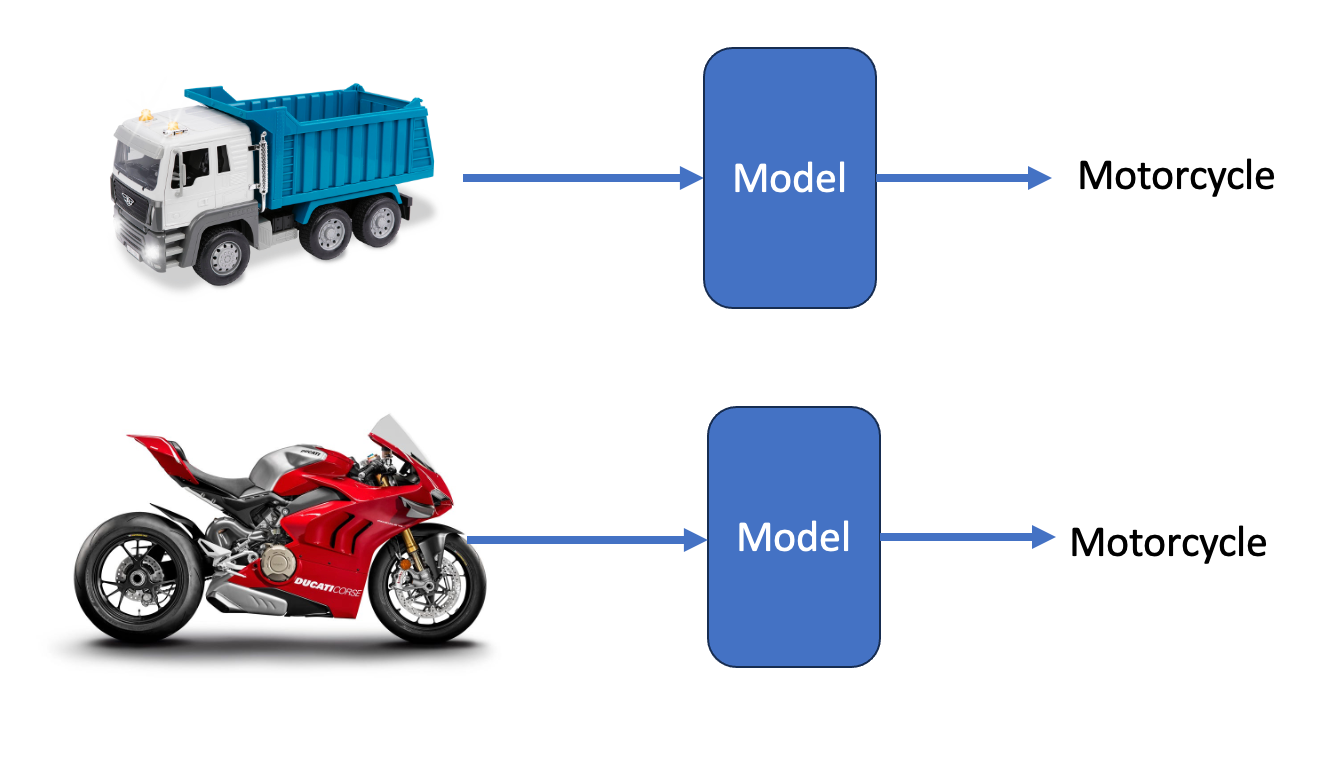Figure 1: The stated figure shows two prediction examples. The first example displays a wrong prediction of the ground truth class - the image shows a truck, but the model classifies it as a motorcycle. The second example displays a correct prediction of the ground truth class.

We initialize the confusion matrix to be a zero matrix with dimensions of (num_classes, num_classes).

For every image, we generate a pair of classification tags with the following format (prediction, ground truth), e.g. (Motorcycle, Truck) and (Motorcycle, Motorcycle) in the above case. For all such pairs, we can count the frequency of each pair and put the total count in the corresponding index of the confusion matrix. In our scenario, a possible confusion matrix might look like this: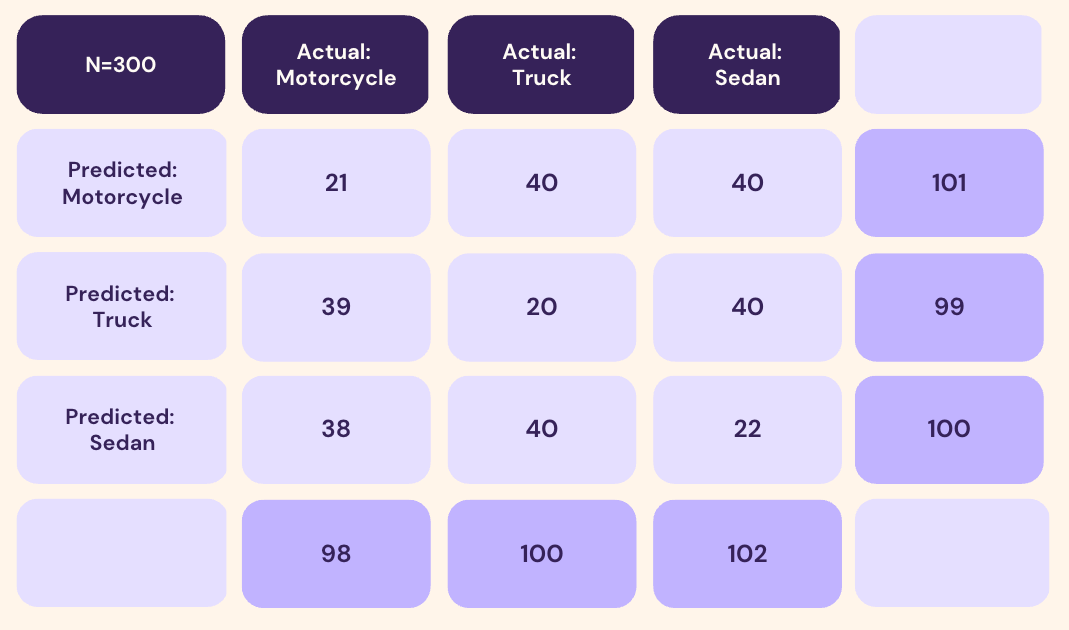Figure 2: Example of a confusion matrix being used to quantify the classification tags with their frequency and total count.

The individual cells represent the number of examples that have the corresponding prediction in that row and the corresponding ground truth in the column. The extra rows and columns indicate the sum of predictions made by the model for each class. In this case, the model made a total of 101 Motorcycle predictions, of which 21 of them are correct and the 80 remaining are false.

As shown above, the confusion matrix is a simple aggregation of frequencies that indicate the distribution over predicted and ground truth classes.

## How Is a Confusion Matrix Computed for Other Computer Vision Tasks?

While confusion matrices are very intuitively calculated from classification models, because their only output is a single class, confusion matrices can become more complicated in terms of their underlying computation for more complex computer vision tasks. Following the computer vision tasks we offer, we will describe how confusion matrices can be calculated for object detection, instance segmentation, and semantic segmentation, grouped by implementation.

#### Object Detection and Instance Segmentation

Object detection and instance segmentation both have the same goal of identifying individual objects. At the object level, the same pairs of (prediction, ground truth) can be constructed as long as one is able to match the ground truth object with a predicted annotation. The main difference between object detection and instance segmentation is the annotation type, where objects are annotated by bounding boxes versus binary masks. As shown below with the image of object detection models creating red prediction boxes on green ground-truth annotations, predictions are not always capable of making accurate predictions, or the same number of predictions, which creates some issues with directly using location on the image to create 1-to-1 pairings between predictions and ground-truths.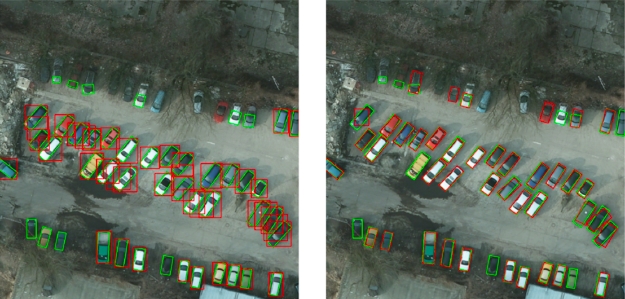Figure 3: Example showcasing object detection whereby red boxes denote the ground-truth annotations while the green boxes depict predictions that were obtained from object detection models like EfficientNet

To construct confusion matrices from binary masks and bounding boxes, we can instead represent these masks as polygons, and thus treat all annotations from ground truth and predictions as polygons. To match polygons between ground truth and prediction, this can be done by calculating the IoU (Intersection over Union for every single pair of (Prediction, Ground-truth) boxes/polygons. We can construct an IoU matrix for predictions and ground truth annotations. From there, to create one-to-one pairings, we take the highest IoU’s as reference as long as they are above a certain threshold. If there are multiple IoU’s of the same value, the match is made at random.Figure 4: An example of an IoU matrix, where a valid IoU is represented by the color green, and a paired IoU is indicated by the color yellow.

As an example, let us imagine that we have an image with three ground truth annotations of three cars and trucks, and the model has made four predictions. In the example above, with a set IoU threshold of 0.6, Car Prediction 2 has a valid IoU with all three ground-truths, but because we want to achieve a one-to-one map between a prediction and a ground truth, we only pair up the highest IoU ground-truth with Car Prediction 2, which is Truck Ground-truth 3. Similarly, with Truck Prediction 3, the only valid ground-truth based on IoU is Truck Ground-truth 1, so we pair them up. For Car Prediction 1 and Truck Prediction 4, they are not getting paired by any ground truth class based on the threshold, so we pair them with the Background class, designating that the model is predicting that there is no object. Additionally, notice that Car Ground-truth 2 does not have a paired prediction despite having a valid prediction by IoU thresholds, as that particular prediction has already been paired with a more fitting ground truth. As such, we assign it to the Background class as well, representing that the model failed to get this object and predict it in none of the classes. The resulting pairs inputted into the confusion matrix are (Truck, Car) from Truck Prediction 3 and Car Ground-truth 1, (Background, Car) from Car Ground-truth 2, and (Car, Truck) from Car Prediction 2 and Truck Ground-truth 3.

#### Semantic Segmentation

Semantic segmentation comes from a differing task perspective than object detection and instance segmentation. Since semantic segmentation models are designed to perform classification for each pixel on the image rather than identify individual objects, we can evaluate the corresponding  confusion matrix by assigning (prediction, ground-truth) pairs per pixel.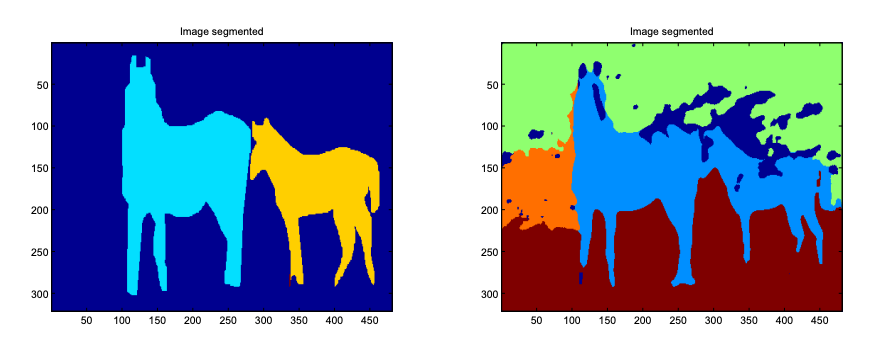Figure 5: The first image displays an example of a ground-truth segmentation, and the second image displays the prediction from a U-Net model

To illustrate, we can use the above example. \text{ConfusionMatrix}_{i, j} represents the number of pixels belonging to any predicted segment which is being labeled as class i and ground-truth segment class j.

## How Does a Confusion Matrix Help with Model Evaluation?

These values can be used to calculate more traditional statistics such as True Positive (TP), True Negative (TN), False Positive (FP), False Negative (FN), Recall, Precision, and F1. They also provide the specific distributions that allow machine learning practitioners to determine common pitfalls such as one specific class like Truck being commonly misclassified as Sedan in the model. In the ideal case, one would hope for a confusion matrix with only non-zero entries along the downward diagonal, meaning that all prediction and ground-truth classes match perfectly. However, in the non-ideal classes, frequencies in other parts of the matrix can reveal insights about the classification issues.

For instance, we can examine the frequency distribution of the Motorcycle class.

In this case, we can derive the following statistics:

• True Positive: This means the model is predicting an image is a Motorcycle when it is a Motorcycle
• False Positive: This means the model is predicting an image is a Motorcycle when it is not
• False Negative: Predictions in this category mean the model predicted a different class than the Motorcycle
• True Negative: This means that the model has correctly predicted it to not be Motorcycle
• Recall: The recall is the ratio: True Positive/(True Positive + False Negative) (the denominator is the number of Motorcycle ground truth) which measures the chance that the model would predict the image as a Motorcycle given it is a Motorcycle.
• Precision: Precision is the ratio: True Positive/(True Positive + False Positive) (the denominator is the total of all Motorcycle predictions) which measures the correctness of the Motorcycle prediction, how many of them are truly correct.

If we were to calculate all these numbers for each class, then average them by the number of classes, we would have two resulting numbers: Macro-averaging Precision and Recall.

This is just a way to get the overall Recall and Precision of the model, there are other ways as well, as long as you have calculated the True Positive, True Negative, and False Negative as in above.

• Micro-averaging: You add up the individual true positives, false positives, and false negatives for each class and then calculate precision and recall (remember that these 2 numbers do not require True Negative in the calculation, which might be recounted multiple times if you are using this way) from those sums. This method gives equal weight to each instance and is useful when your classes are imbalanced.
• Weighted averaging: Similar to macro-averaging, you weight the precision or recall scores by the number of actual instances for each class before taking the average. This accounts for class imbalance by giving more weight to the larger classes.

Each of these statistics have their own different strengths and weaknesses, and their relevance is usually based on the context of the task. For example, for anomaly detection in a manufacturing line, in which anomalies are considered positive classifications, the goal of a classifier or object detector would be to reduce false negatives, and thus, increase recall, because letting anomalies slip through is far worse as it reduces than categorizing acceptable products as anomalies, which incur small costs.

## How to Use Nexus’ Confusion Matrix

Nexus’ Confusion Matrix can be used as another form of evaluation during training, alongside other features like our real-time training dashboard and Advanced Evaluation.  During training, the Confusion Matrix tab can be found at the top of the training dashboard, in the Run page, as shown below.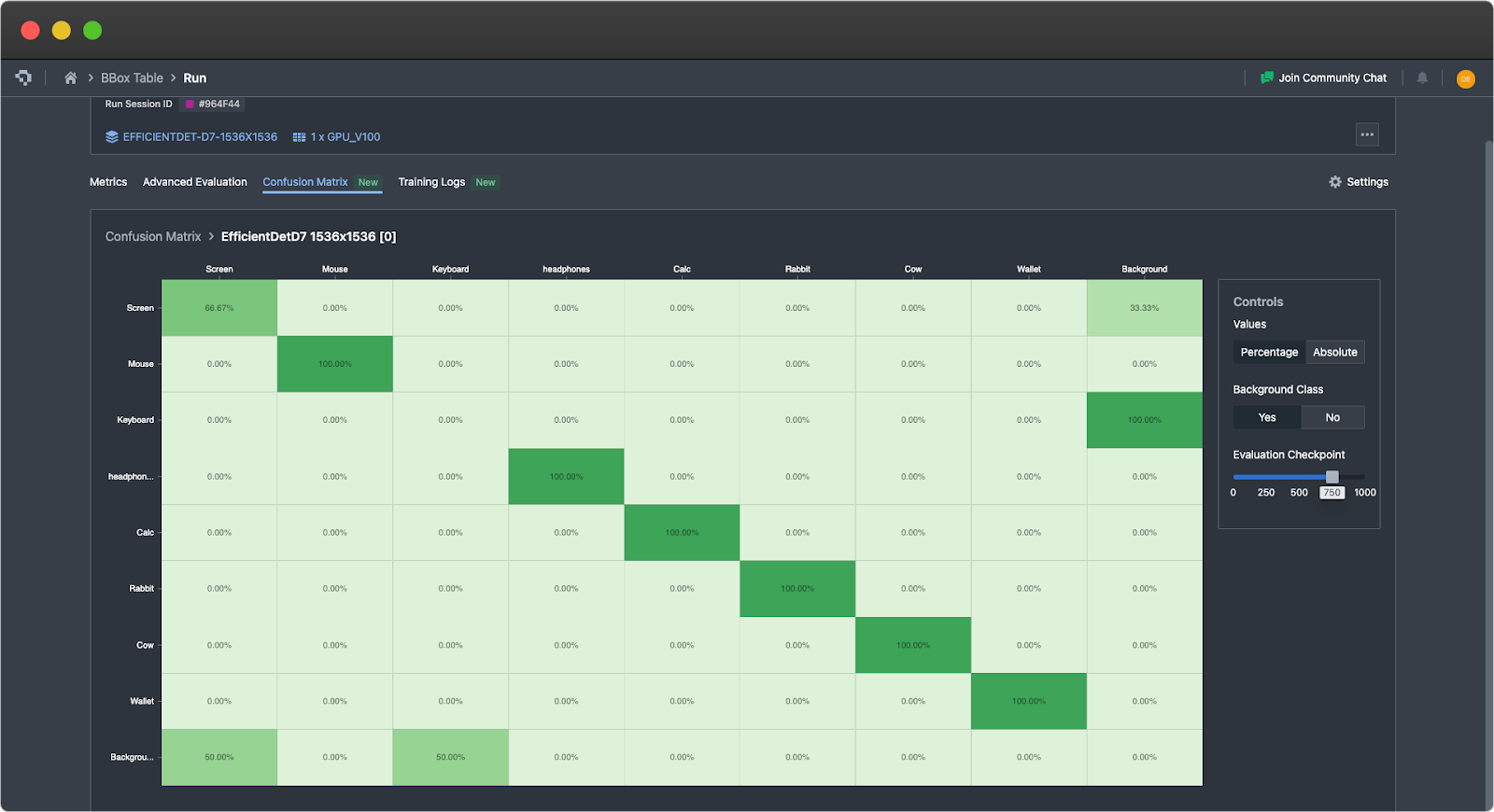In the Run page, the Confusion Matrix tab can be found at the top of the training dashboard

Our confusion matrix is computed in exactly the methods described above, with ground truth classes being represented as columns, and the prediction classes represented as rows. To aid in ease of interpretability, it uses color gradients to highlight differences in distributions, where lighter colors represent low proportions and darker colors represent higher proportions. To improve the user experience, we’ve also added a few options to improve interpretability. In the default view, the background class entries are omitted, but these can be toggled with ‘Yes’ or ‘No’ buttons under Background Class.

Additionally, in the default view, the confusion matrix is normalized based on ground truth, meaning that the numbers are percentages calculated row-wise. This means that the sum of all the entries over the row is 100%. These normalized values provide the proportion of confusion to other classes for each model’s predicted class, as well as the precision per class along the diagonal. This will automatically update to include or not include the background class, depending on whether it is toggled on or off. The percentage view allows users to more easily perceive the distribution of results that can occur. One can still view the raw computed values before normalizing by selecting Absolute. This will allow users to view and verify the underlying values.

Finally, similar to Advanced Evaluation, one can scroll across the evaluation checkpoints to see how the confusion matrix for the model has evolved. Ideally, one should observe that the proportions for each row should converge towards the downward diagonal, such that prediction and ground truth classes are maximally aligned.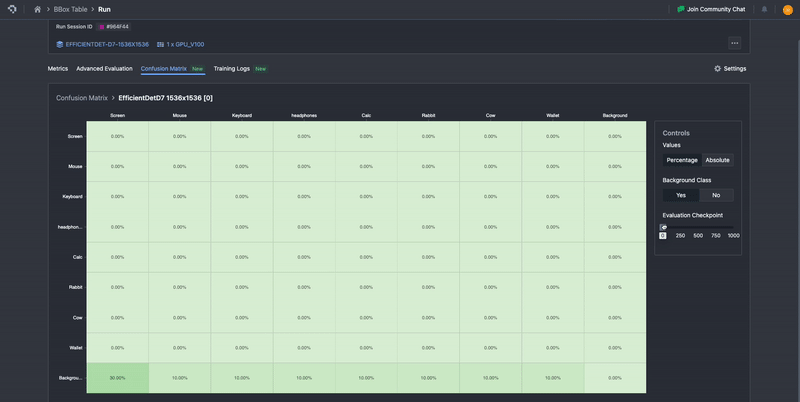Scroll across the evaluation checkpoints to see how the confusion matrix for the model has evolved

### What’s Next?

Nexus offers other features that help users better understand different parts of the training process. At the dataset level, you can use these tools such as heatmaps and tag distribution graphs to better understand your dataset. For augmentation setups, you can preview augmentations on the Workflow page to check whether the augmentations emulate the environments you want to artificially simulate. Finally, during the training process, you can also use training graphs and Advanced Evaluation to track the performance of your training in real time.